Chapter 10: Liquid Extraction¶

Ex10.1: Page 494¶

In :
# Illustration 10.1
# Page: 494

print'Illustration 10.1 - Page: 494\n\n'

# solution
import matplotlib.pyplot as plt
%matplotlib inline
import pylab
#****Data****#
# a:water b:isopropyl ether c:acetic acid
xF = 0.30;# [mol fraction]
yS = 0;# [mol fraction]
S1 = 40.0;# [kg]
B1 = 40.0;# [kg]
#*******#

# Equilibrium data at 20 OC:
# Wa: Wt. percent of a
# Wb: Wt. percent of b
# Wc: Wt. percent of c
# Data1 = [Wc Wa Wb]
# Data1: water layer
Data1 = numpy.array([(0.69 ,98.1, 1.2),(1.41, 97.1 ,1.5),(2.89 ,95.5 ,1.6),(6.42 ,91.7 ,1.9),(13.30, 84.4, 2.3),(25.50 ,71.1 ,3.4),(36.70 ,58.9 ,4.4),(44.30 ,45.1 ,10.6),(46.40 ,37.1 ,16.5)])
# Data2: isopropyl ether layer
Data2 = numpy.array([(0.18 ,0.5 ,99.3),(0.37, 0.7 ,98.9),(0.79, 0.8, 98.4),(1.93 ,1, 97.1),(4.82, 1.9, 93.3),(11.40, 3.9, 84.7),(21.60, 6.9, 71.5),(31.10, 10.8, 58.1),(36.20 ,15.1 ,48.7)])

plt.plot((Data1[:,2])/100,(Data1[:,0])/100,label="x Vs fraction ether")
plt.plot((Data2[:,2])/100,(Data2[:,0])/100,label="y Vs fraction ether")
plt.grid('on');
plt.legend(loc='lower center');
ax=pylab.gca()
ax.set_xlabel("Wt fraction of isopropyl ether");
ax.set_ylabel("Wt fraction of acetic acid");
plt.ylim((0,0.3))
plt.xlim((0,1))
plt.show();
# x: Wt fraction of acetic acid in water layer.
# y: Wt fraction of acetic acid in isopropyl layer.

# The rectangular coordinates of Fig 10.9(a) will be used but only upto x = 0.30

# Stage 1:
F = 100;# [kg]
# From Eqn. 10.4:
M1 = F+S1;# [kg]
# From Eqn. 10.5:
xM1 = ((F*xF)+(S1*yS))/M1;
# From Fig. 10.15 (Pg 495):
# Point M1 is located on the line FB and with the help of tie line passing through M1:
x1 = 0.258;# [mol fraction]
y1 = 0.117;# [mol fraction]
# From Eqn. 10.8:
E1 = (M1*(xM1-x1)/(y1-x1));# [kg]
# From Eqn. 10.4:
R1 = M1-E1;# [kg]

# Stage 2:
S2 = 40;# [kg]
B2 = 40;# [kg]
# From Eqn. 10.15:
M2 = R1+B2;# [kg]
# From Eqn. 10.16:
xM2 = ((R1*x1)+(S2*yS))/M2;
# Point M2 is located on the line R1B and the tie line passing through R2E2 through M2:
x2 = 0.227;
y2 = 0.095;
# From Eqn. 10.8:
E2 = (M2*(xM2-x2)/(y2-x2));# [kg]
# From Eqn. 10.4:
R2 = M2-E2;# [kg]

# Stage 3:
S3 = 40;# [kg]
B3 = 40;# [kg]
# From Eqn. 10.15:
M3 = R2+B3;# [kg]
# From Eqn. 10.16:
xM3 = ((R2*x2)+(S3*yS))/M3;
# Point M3 is located on the line R2B and the tie line passing through R3E3 through M3:
x3 = 0.20;# [mol fraction]
y3 = 0.078;# [mol fraction]
# From Eqn. 10.8:
E3 = (M3*(xM3-x3)/(y3-x3));# [kg]
# From Eqn. 10.4:
R3 = M3-E3;# [kg]
Ac = x3*R3;
print"The composited extract is",round((E1+E2+E3),2)," kg\n"
print"The acid content is ",round(((E1*y1)+(E2*y2)+(E3*y3)),2)," kg\n"
print"\n"

# If an extraction to give the same final raffinate concentration were to be done in single stage, the point M would be at the intersection of tie line R3E3 and the line BF.
x = 0.20;# [mol fraction]
xM = 0.12;# [mol fraction]
# From Eqn. 10.6:
S = F*(xF-xM)/(xM-yS);# [kg]
print round(S,2),"kg of solvent would be recquired if the same final raffinate concentration were to be obtained with one stage.\n"
Illustration 10.1 - Page: 494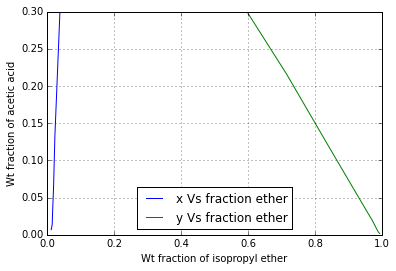The composited extract is 135.05  kg

The acid content is  13.01  kg

150.0 kg of solvent would be recquired if the same final raffinate concentration were to be obtained with one stage.

Ex10.2: Page 497¶

In :
# Illustration 10.2
# Page: 497

print'Illustration 10.2 - Page: 497\n\n'

print'Illustration 10.2 (a)\n\n'

# solution (a)
import matplotlib.pyplot as plt
%matplotlib inline
import pylab

#****Data****#
# a:water b:kerosene c:Nicotine
xF = 0.01;# [wt fraction nicotine]
F = 100.0;# [kg]
B = 150.0;# [kg]
#******#

# Equilibrium data:
# x_prime = kg nicotine/kg water
# y_prime = kg nicotine/kg kerosene
# Data = [x_prime y_prme]
Data = numpy.array([[0 ,0],[0.001011 ,0.000807],[0.00246, 0.001961],[0.00502, 0.00456],[0.00751, 0.00686],[0.00998 ,0.00913],[0.0204, 0.01870]])
xF_prime = xF/(1-xF);# kg nicotine/kg water
A = F*(1-xF);# [kg]
AbyB = A/B;

def f64(x):
return -AbyB*(x-xF)
x = numpy.arange(0,0.01+0.001,0.001);
plt.plot(Data[:,0],Data[:,1],label="Equilibrium line")
plt.plot(x,f64(x),label="Operating Line");
plt.grid('on');
legend(loc='upper left');
plt.xlabel("kg nicotine / kg water");
plt.ylabel("kg nicotine / kg kerosene");
plt.title("Solution 10.2(a)")
plt.show()
# The operating line and equilibrium line intersect at:
x1_prime = 0.00425;# [kg nicotine/kg water]
y1_prime = 0.00380;# [kg nicotine/kg water]
extract = A*(0.01011-x1_prime);
print extract*100,"% of nicotine is extracted.\n\n"

print'Illustration 10.2 (b)\n\n'

# Solution (b)
B = 50.0;# [kg]
# For each stage:
AbyB = A/B;
def f65(x1):
return  -AbyB*(x1-xF)
x1 = numpy.arange(0,0.01+0.001,0.001)
def f66(x2):
return -AbyB*(x2-0.007)
x2 = numpy.arange(0,0.01+0.001,0.001)
def f67(x3) :
return -AbyB*(x3-0.005)
x3 =numpy.arange(0,0.01+0.001,0.001)

plot(Data[:,0],Data[:,1],label="Equilibrium line")
plt.plot(x1,f65(x1),label="Operating Line from xF")
plt.plot(x2,f66(x2),label="Operating Line from 0.007")
plt.plot(x3,f67(x3),label="Operating Line from 0.005")
plt.grid('on');
legend(loc="upper right");
plt.xlim((0,0.012))
plt.ylim((0, 0.010))
plt.xlabel("kg nicotine / kg water");
plt.ylabel("kg nicotine / kg kerosene");
plt.title("Solution 10.2(b)")
plt.show()
# The final raffinate composition:
x3_prime = 0.0034;# [kg nicotine/kg water]
extract = A*(0.01011-x3_prime);
print extract*100," % of nicotine is extracted.\n"
Illustration 10.2 - Page: 497

Illustration 10.2 (a)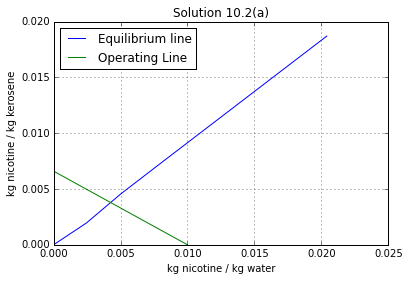58.014 % of nicotine is extracted.

Illustration 10.2 (b)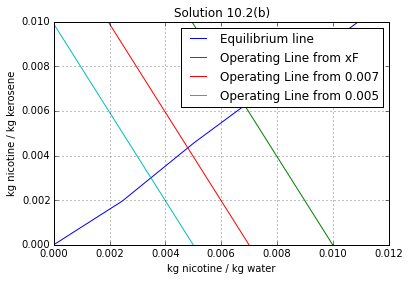66.429  % of nicotine is extracted.

Ex10.3: Page 502¶

In :
# Illustration 10.3
# Page: 502

print'Illustration 10.3 - Page: 502\n\n'

# Solution

import matplotlib.pyplot as plt
%matplotlib inline
import pylab
#****Data****#
# a:water b:isopropyl ether c:acetic acid
F = 8000;# [kg/h]
xF = 0.30;# [wt. fraction acetic acid]
#*******#

# From Illustration 10.1 (Pg 494)
# Equilibrium Data:
# Eqb = [y_star*100 x*100]
Eqb = numpy.array([[0.18 ,0.69],[0.37 ,1.41],[0.79 ,2.89],[1.93, 6.42],[4.82, 13.30],[11.40, 25.50],[21.60 ,36.70],[31.10 ,44.30],[36.20, 46.40]]);

# Solution(a)

# From Figure 10.23 (Pg 503):
# For minimum solvent rate:
y1 = 0.143;# [Wt fraction of acetic acid in isopropyl ether layer]
xM = 0.114;# [Wt fraction of acetic acid in water layer]
# From Eqn. 10.24:
Bm = (F*xF/xM)-F;# [kg/h]
print"Minimum solvent rate: ",Bm," kg/h\n"
print"\n"

# Solution (b)

B = 20000.0;# [kg solvent/h]
yS = 0;
S = B;
# From Eqn 10.24:
xM = ((F*xF)+(S*yS))/(F+S);
# From Fig. 10.23 (Pg 503):
y1 = 0.10;
# Operating curve data:
# Operat = [YsPlus1 Xs]
Operat = numpy.array([[0 ,0.02],[0.01 ,0.055],[0.02 ,0.09],[0.04 ,0.150],[0.06, 0.205],[0.08, 0.250],[0.1 ,0.3]]);

plt.plot(Eqb[:,1]/100,Eqb[:,0]/100,label="Operating Line")
plt.plot(Operat[:,1],Operat[:,0],label="Equilibrium Line")
plt.grid('on');
plt.ylim((0,y1));
plt.xlim((0,xF));
legend(loc='upper left');
plt.xlabel("Wt. fraction acetic acid in water solution");
plt.ylabel("Wt. fraction acetic acid in isopropyl ether solution");
plt.title("Solution 10.3")
plt.show()
# From Figure scf(22):
xNp = 0.02;
Np = 7.6;
# By acid balance:
M = B+F;
E1 = M*(xM-xNp)/(y1-xNp);# [kg/h]
RNp = M-E1;# [kg/h]
print"Number of theoretical Stages: \n",Np
print"Weight of the extract:",E1,"kg/h\n"
print"Weight of the raffinate ",RNp," kg/h\n",
Illustration 10.3 - Page: 502

Minimum solvent rate:  13052.6315789  kg/h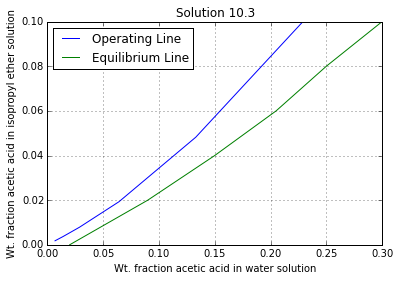Number of theoretical Stages:
7.6
Weight of the extract: 23000.0 kg/h

Weight of the raffinate  5000.0  kg/h

Ex10.4: Page 506¶

In :
# Illustration 10.4
# Page: 506

print'Illustration 10.4 - Page: 506\n\n'

import matplotlib.pyplot as plt
%matplotlib inline
import pylab
import numpy

# Solution

#****Data****#
# a:water b:kerosene c:Nicotine
F = 1000.0;# [kg/h]
xF = 0.01;# [wt. fraction acetic acid]
#*******#

# Equilibrium data:
# x_prime = kg nicotine/kg water
# y_prime = kg nicotine/kg kerosene
# Eqb = [x_prime y_prme]
Eqb = numpy.array([[0 ,0],[0.001011, 0.000807],[0.00246, 0.001961],[0.00502 ,0.00456],[0.00751, 0.00686],[0.00998, 0.00913],[0.0204 ,0.01870]]);

# Solution (a)

A = 1000*(1-xF);# [kg water/h]
yS = 0;
yS_prime = 0;
y1_prime = 0;
xF_prime = xF/(1-xF);# [kg nicotine/kg water]
# For xF_prime = 0.0101:
yk = 0.0093;
xNp = 0.001;# [wt. fraction acetic acid]
xNp_prime = xNp/(1-xNp);# [kg nicotine/kg water]
# For infinite stages:
# Operating Line should pass through (xNp_prime,y1_prime) & (xF_prime,yk)
Operat = numpy.array([[xNp_prime, y1_prime],[xF_prime ,yk]]);

plt.plot(Eqb[:,0],Eqb[:,1],label="equilibrium Line")
plt.plot(Operat[:,0],Operat[:,1],label="Operating Line")
plt.grid('on');
legend(loc='upper left');
plt.xlabel("kg nicotine / kg water");
plt.ylabel("kg nicotine / kg kerosene");
plt.title("Solution 10.4(a)")
plt.xlim((0,0.012))
plt.ylim((0,0.01))
plt.show()
AbyBm = (yk-y1_prime)/(xF_prime-xNp_prime);
Bm = A/AbyBm;# [kg kerosene/h];
print"Mininmum kerosene rate: ",round(Bm,2)," kg kerosene/h \n"

# Solution (b)

B = 1150.0;# [kg/h]
AbyB = A/B;
# From Eqn. 10.36:
y2_prime = ((xF_prime-xNp_prime)*AbyB)+yS_prime;# [kg nicotine/kg kerosene]
# Operating Line should pass through (xNp_prime,y1_prime) & (xF_prime,y2_prime)
Operat = numpy.array([[xNp_prime, y1_prime],[xF_prime, y2_prime]]);

plt.plot(Eqb[:,0],Eqb[:,1],label="equilibrium Line")
plt.plot(Operat[:,0],Operat[:,1],label="Operating Line")
plt.grid('on');
plt.legend(loc='upper left');
plt.xlabel("kg nicotine/kg water");
plt.ylabel("kg nicotine/kg kerosene");
plt.title("Solution 10.4(b)")
plt.xlim((0,0.012))
plt.ylim((0,0.01))
plt.show()
# From Figure:
Np = 8.3;
print"Number of theoretical stages: \n",Np
Illustration 10.4 - Page: 506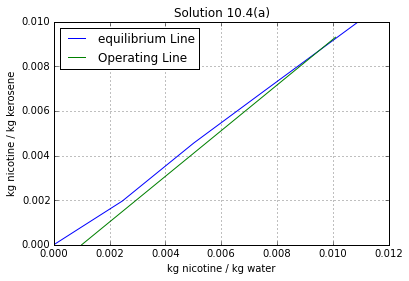Mininmum kerosene rate:  968.71  kg kerosene/h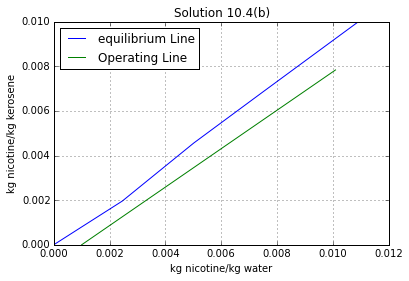Number of theoretical stages:
8.3

Ex10.5: Page 510¶

In :
# Illustration 10.5
# Page: 510

print'Illustration 10.5 - Page: 510\n\n'

# solution

import matplotlib.pyplot as plt
%matplotlib inline
import pylab
import numpy.linalg as lin
import numpy

#****Data****#
# a:ethylbenzne b:diethylene glycol c:styrene
F = 1000;# [kg/h]
xF = 0.5;# [Wt. fraction styrene]
xPE = 0.9;# [kg styrene/kg hydrocarbon]
xRNp = 0.1;# [kg styrene/kg hydrocarbon]
#******#

# X: kg styrene/kg hydrocarbon
# Y: kg styrene/kg hydrocarbon
# N:kg glycol/kg hydrocarbon
# Equilibrium data:
# Hydrocarbon rich solutions:
# Eqb1 = [X N]
Eqb1 = numpy.array([[0 ,0.00675],[0.0870 ,0.00817],[0.1833, 0.00938],[0.288 ,0.01010],[0.384 ,0.01101],[0.458, 0.01215],[0.464 ,0.01215],[0.561 ,0.01410],[0.573, 0.01405],[0.781 ,0.01833],[1 ,0.0256]]);
# Solvent rich solutions:
# Eqb2 = [Y_star N]
Eqb2 = numpy.array([[0 ,8.62],[0.1429 ,7.71],[0.273, 6.81],[0.386, 6.04],[0.480, 5.44],[0.557, 5.02],[0.565, 4.95],[0.655, 4.46],[0.674, 4.37],[0.833, 3.47],[1 ,2.69]]);

plt.plot(Eqb1[:,0],Eqb1[:,1],label="X Vs N")
plt.plot(Eqb2[:,0],Eqb2[:,1],label="Y Vs N")
plt.grid('on');
legend(loc='upper right');
plt.xlabel("kg styrene / kg hydrocarbon");
plt.ylabel("kg diethylene glycol / kg hydrocarbon");
plt.title("Equilibrium Data")
# In Fig. 10.31 (Pg 512):
# Point E1 is located.
NE1 = 3.10;

# solution (a)

# From Fig. 10.30 (Pg 511):
Np = 9.5;
print"Minimum number of theoretical stages:\n",Np
print"\n"

# Solution (b)

# The tie line when extended passes through F provides the minimum reflux ratio.
# From the plot:
N_deltaEm = 20.76;
# From Eqn. 10.48:
Ratiom = (N_deltaEm-NE1)/NE1;# [kg reflux/kg extract product]
print"Minimum extract reflux ratio: ",round(Ratiom,3),"kg reflux/kg extract product\n"
print"\n"

# Solution (c)

Ratio = 1.5*Ratiom;# [kg reflux/kg extract product]
# From Eqn. 10.48;
N_deltaE = (Ratio*NE1)+NE1;
# Point deltaE is plotted.
# A straight line from deltaE through F intersects line X = 0.10 at deltaR.
N_deltaR = -29.6;
# In Fig. 10.31 (Pg 512):
# Random lines are drawn from deltaE for the concentrations to the right of F, and from deltaR for those to the left,and intersection of these with the solubility curves provide the coordinates of the opeating curve.
# The tie line data are plotted directly to provide the equilibrium curve.
# From Fig. 10.32 (Pg 513):
Np = 15.5;
# Feed is to be introduced in the seventh from the extract product end of cascade.
# From Fig. 10.31 (Pg 512):
XRNp = 0.10;
NRNp = 0.0082;
# Basis:1 hour.
# Overall plant balance:
# (1): PE_prime+RNp_prime = F
# C Balance
# (2): PE_prime*(1-XRNp)+RNp_prime*XRNp = F*xF
# Solving (1) & (2) simultaneously:
a = numpy.array([[1 ,1],[(1-XRNp), XRNp]]);
b = numpy.array([F,F*xF]);
soln =lin.solve(a,b)
PE_prime = soln;# [kg/h]
RNp_prime = soln;# [kg/h]
RO_prime = Ratio*PE_prime;# [kg/h]
# From Eqn 10.39:
E1_prime = RO_prime+PE_prime;# [kg/h]
BE = E1_prime*NE1;# [kg/h]
E1 = BE+E1_prime;# [kg/h]
RNp = RNp_prime*(1+NRNp);# [kg/h]
S = BE+(RNp_prime*NRNp);# [kg/h]
print"Number of theoretical stages: \n",Np
print"Extract Flow Rate: ",round(E1,2)," kg/h\n"
print"solvent Flow Rate: ",S," kg/h\n"
#the answers are slightly different in textbook due to approximation while here answers are precise
Illustration 10.5 - Page: 510

Minimum number of theoretical stages:
9.5

Minimum extract reflux ratio:  5.697 kg reflux/kg extract product

Number of theoretical stages:
15.5
Extract Flow Rate:  19567.58  kg/h

solvent Flow Rate:  14799.1  kg/h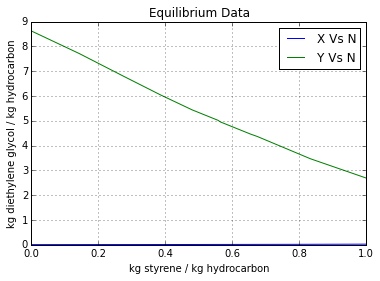Ex10.6: Page 516¶

In :
# Illustration 10.6
# Page: 516

print'Illustration 10.6 - Page: 516\n\n'

import numpy.linalg as lin
import matplotlib.pyplot as plt
%matplotlib inline

# solution

#****Data****#
# a:heptane b:p-chloronitrobenzene c:o-chloronitrobenzene d:aq. methanol
xb = 0.4;# [Wt fraction]
xC = 0.60;# [Wt fraction]
F = 100;# [kg]
# The para isomer(b) favours the heptane(a) and the ortho isomer(c) favours the methanol(d).
# Basis: 1 hour.
A = 2400;# [kg/h]
D = 2760;# [kg/h]
xbW = 0.8;# [Wt fraction]
xbZ = 0.15;# [Wt fraction]
kb=1.35;
kc=0.835;
#*******#

B = xb*F;# [kg]
C = F-B;# [kg]
# W = kg A rich product, after solvent removal
# Z = kg D rich product, after solvent removal
# B balance:
# (1): (0.80*W)+(0.15*Z) = B
# C balance:
# (2): (0.20*W)+(0.85*Z) = C
# Solving (1) & (2) simultaneously:
a = numpy.array([[0.80, 0.15],[0.20, 0.85]]);
b = [B,C];
soln = lin.solve(a,b)
W = soln;
Z = soln;
Wb = xbW*W;# [kg]
Wc = W-Wb;# [kg]
Zb = xbZ*Z;# [kg]
Zc = Z-Zb;# [kg]
xB1_prime = Zb/D;
xC1_prime = Zc/D;
yB1_prime = Wb/D;
yC1_prime = Wc/D;
DbyA = D/A;
# Equilibrium curve:
# First distribution coeffecient: yB_star/xB_prime = 1.35
def  f68(x1):
return  kb*x1
x1 = numpy.arange(0,0.06+0.01,0.01)
# Second distribution coeffecient: yC_star/xC_prime = 0.835
def  f69(x2):
return kc*x2
x2 = numpy.arange(0,0.06+0.01,0.01)
# Operating Line, corresponding to First distribution coeffecient:
def f70(x3):
return (DbyA*x3)+yB1_prime
x3 = numpy.arange(0,0.06+0.01,0.01)
def f71(x4):
return DbyA*(x4-xB1_prime)
x4 = numpy.arange(0,0.06+0.01,0.01)
# Operating Line, corresponding to Second distribution coeffecient:
def f72(x5):
return (DbyA*x5)+yC1_prime
x5 = numpy.arange(0,0.06+0.01,0.01)
def  f73(x6):
return (DbyA)*(x6-xC1_prime);
x6 = numpy.arange(0,0.06+0.01,0.01)

plot(x1,f68(x1),label="Equilibrium curve")
plt.plot(x3,f70(x3),label="Operating curve")
plt.plot(x4,f71(x4),label="Operating curve");
plt.grid('on');
plt.legend(loc='upper left');
plt.xlabel("xB_prime");
plt.ylabel("yB_prime");
plt.title("yB_star/xB_prime = 1.35");
plt.xlim((0,0.05))
plt.ylim((0,0.07))
plt.show()

plot(x2,f69(x2),label="Equilibrium curve")
plt.plot(x5,f72(x5),label="Operating curve")
plt.plot(x6,f73(x6),label="Operating curve")
plt.grid('on');
plt.legend(loc='upper left');
plt.xlabel("xC_prime");
plt.ylabel("yC_prime");
plt.title("yC_star/xC_prime = 0.835");
plt.xlim((0,0.06))
plt.ylim((0,0.07))
plt.show()
# The stages are constructed.
# The feed matching is shown on Fig. 10.37 (Pg 518):
f_prime = 6.6;
fstage = 4.6;
print"Number of ideal stage is \n",fstage+f_prime-1
print"The feed stage is ",fstage,"th from the solvent-D inlet\n"
Illustration 10.6 - Page: 516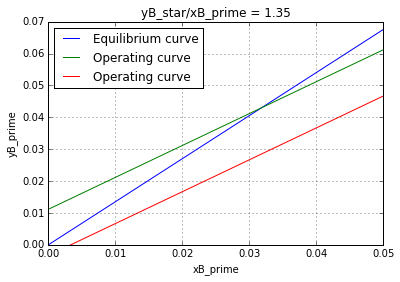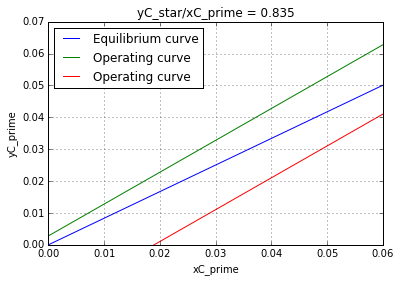Number of ideal stage is
10.2
The feed stage is  4.6 th from the solvent-D inlet

Ex10.7: Page 525¶

In :
# Illustration 10.7
# Page: 525

print'Illustration 10.7 - Page: 525\n\n'
# solution

import math
#****Data****#
# c:Water d:Toulene
Density_c = 998;# [kg/cubic m]
viscosity_c = 0.95*10**(-3);# [kg/m.s]
Dc = 2.2*10**(-9);# [square m/s]
Density_d = 865;# [kg/cubic m]
viscosity_d = 0.59*10**(-3);# [kg/m.s]
Dd = 1.5*10**(-9);# [square m/s]
sigma = 0.022;# [N/m]
Dist = 20.8;# [Distribution Coeffecient]
d = 0.5;# [m]
h = 0.5;# [m]
di = 0.15;# [m]
N = 13.3;# [r/s]
g = 9.81;# [m/s^2]
qC = 3*10**(-3);# [cubic m/s]
qD = 3*10**(-4);# [cubic m/s]
#********#

V = math.pi*h*d**2/4;# [Vessel volume,cubic m]
phi_DF = qD/(qD+qC);# [Volume fraction toulene]
# Assume:
phi_Dbyphi_DF = 0.9;
phi_D = phi_Dbyphi_DF*phi_DF;
phi_W = 1-phi_D;
# From Eqn. 10.56:
Density_M = (Density_c*phi_W)+(Density_d*phi_D);# [kg/cubic m]
if phi_W>0.4:
viscosity_M = (viscosity_c/phi_W)*(1+(6*viscosity_d*phi_D/(viscosity_d+viscosity_c)));# [kg/m s]
else:
viscosity_M = (viscosity_c/phi_D)*(1-(1.5*viscosity_c*phi_W/(viscosity_d+viscosity_c)));# [kg/m s]

# Impeller Reynold's Number:
IRe = (di**2*N*Density_M/viscosity_M);
# From Fig 6.5 (Pg 152), curve g:
Po = 0.72;
P = Po*Density_M*N**3*di**5;# [W]
# From Eqn. 10.61:
Value1 = P*qD*viscosity_c**2/(V*sigma**3);
Value2 = viscosity_c**3/(qD*Density_c**2*sigma);
Value3 = Density_c/(Density_c-Density_d);
Value4 = sigma**3*Density_c/(viscosity_c**4*g);
Value5 = viscosity_d/viscosity_c;
phi_Dbyphi_DF = 3.39*Value1**0.247*Value2**0.427*Value3**0.430*Value4**0.401*Value5**0.0987;
# The value of phi_Dbyphi_DF is sufficiently close to the value 0.90 assumed earlier.
phi_D = phi_Dbyphi_DF*phi_DF;
# From Eqn. 10.6:
Value6 = viscosity_c/Density_c;# [square m/s]
Value7 = P/(V*Density_M);
Value8 = sigma/Density_c;
dp = 10**(-2.066+(0.732*phi_D))*Value6**0.0473*Value7**(-0.204)*Value8**(0.274);# [m]
a = 6*phi_D/dp;# [square m/cubic m]
Sca = viscosity_c/(Density_c*Dc);
# From Eqn. 10.65:
Shc = 65.3;
kLc = Shc*Dc/dp;# [kmol/square m s (kmol/cubic m)]
thetha = V/(qD+qC);# [s]
# From Table 10.1 (Pg 524):
# lambda = [lambda1 lambda2 lambda3]
Lambda = [1.359 ,7.23, 17.9];
# B = [B1 B2 B3]
B = [1.42 ,0.603 ,0.317];
Val = numpy.zeros(3);
Sum = 0;
for n in range(0,3):
Val[n] = (B[n]**2)*exp((-Lambda[n])*64*Dd*thetha/dp**2);
Sum = Sum+Val[n];

# From Eqn. 10.66:
kLd = -(dp/(6*thetha))*math.log((3.0/8)*Sum);
mCD = 1.0/Dist;
# From Eqn. 10.67:
KLd = 1/((1/kLd)+(1/(mCD*kLc)));# [kmol/square m s (kmol/cubic m)]
Z = 0.5;# [m]
Vd = qD/(math.pi*Z**2.0/4);# [m/s]
# From Eqn.10.70:
NtoD = Z/(Vd/(KLd*a));
# From Eqn. 10.71:
EMD = NtoD/(NtoD+1);
print"Expected stage efficiency: \n",round(EMD,2)
Illustration 10.7 - Page: 525

Expected stage efficiency:
0.93

Ex10.8: Pg-539¶

In :
# Illustration 10.8
# Page: 539

print'Illustration 10.8 - Page: 539\n\n'

# solution
import math
from scipy.optimize import fsolve

#****Data****#
# a:acetic acid c:Water d:Isopropylether layer
# Water solution (continuous):
C = 8000.0;# [kg/h]
xCn = 0.175;# [mass fraction]
Density_c = 1009.0;# [kg/cubic m]
viscosity_c = 3.1*10**(-3);# [kg/m.s]
Dc = 1.24*10**(-9);# [square m/s]

# Isopropyl Ethr Layer:
D = 20000.0;# [kg/h]
xDnPlus1 = 0.05;# [mass fraction]
Density_d = 730;# [kg/cubic m]
viscosity_d = 0.9*10**(-3);# [kg/m.s]
Dd = 1.96*10**(-9);# [square m/s]

sigma = 0.013;# [/N/m]
m = 2.68;# [Distributon coeffecient]
#*******#

Ma = 60.1;
g = 9.81;# [m/square s]
cCn = xCn*Density_c/Ma;# [kmol/cubic m]
cDnPlus1 = xDnPlus1*Density_d/Ma;# [kmol/cubic m]
mCD = m*(Density_c/Density_d);# [(kmol/cubic min ether)/(kmol/cubic m in water)]

# Perforations:
Do = 0.006;# [m]
pitch = 0.015;# [m]
qD = D/(3600.0*Density_d);# [cubic m/s]
delta_Density = Density_c-Density_d;# [kg/cubic m]
Value1 = Do/(sigma/(delta_Density*g))**0.5;
if Value1<0.1785:
# From Eqn. 10.74(a):
doBydj = (0.485*Value1**2)+1;
else:
# From Eqn. 10.74(b)
doBydj = (1.51*Value1)+0.12;

dj = Do/doBydj;# [m]
Vomax = 2.69*((dj/Do)**2)*(sigma/(dj*((0.5137*Density_d)+(0.4719*Density_c))))**0.5;# [m/s]
# Since Vomax is less than 0.1:
Vo = 0.1;# [m/s]
Ao = qD/Vo;# [square m]
No = Ao/(math.pi*Do**2.0/4);# [square m]
# From Eqn. 6.30:
# Plate area for perforation:
Aa = Ao/(0.907*(Do/pitch)**2);# [square m]

# Downspout:
dp = 0.0007;# [m]
# From Eqn. 10.75:
U = Density_c**2*sigma**3/(g*viscosity_c**4*delta_Density);
# From Fig. 10.47 (Pg 534):
ordinate = 1.515;
abcissa = 0.62;
def f74(Vt):
return   abcissa-(dp*Vt*Density_c/(viscosity_c*U**0.15))
Vt = fsolve(f74,7);# [m/s]
Vd = Vt;# [m/s]
qC = C/(Density_c*3600);# [cubic m/s]
# From Table 6.2 (Pg 169):
# Allowing for supports and unperforated area:
At = Aa/0.65;# [square m]
T = (At*4/math.pi)**0.5;# [m]

# Drop Size:
alpha1 = 10.76;
alpha2 = 52560;
alpha3 = 1.24*10**6;
alpha4 = 3.281;
abcissa = (alpha2*sigma*Do/delta_Density)+(alpha3*Do**1.12*Vo**0.547*viscosity_c**0.279/delta_Density**1.5);
Parameter = alpha1*Density_d*Vo**2/(delta_Density);
ordinate = 0.024;
dp = ordinate/alpha4;

# Coalesced layer:
Vn = qD/An;# [m/s]
# From Eqn. 10.80:
ho = (Vo**2-Vn**2)*Density_d/(2*g*0.67**2*delta_Density);# [m]
hD = ho;
# From Eqn. 10.82:
hC = 4.5*Vd**2*Density_c/(2*g*delta_Density);# [m]
# From Eqn. 10.78:
h = hC+hD;
# Since this is very shallow, increase it by placing an orifice at the bottom of the downspout.
# VR: Velocity through the restriction.
# hR: Corresponding depth of the coalesced layer.
# Assume:
Vr = 0.332;# [m/s]
hr = (Vr**2-Vd**2)*Density_c/(2*0.67**2*delta_Density);
Ar = qC/Vr;# [square m]
dr = (4*Ar/math.pi)**0.5;# [m]
h = h+hr;# [m]
# The above results are satisfacyory.
Z = 0.35;# [m]
# Lead the downspout apron to within 0.1 m of the tray below.

# Dispersed-phase holdup:
# From Eqn. 10.48:
Vsphi_D = Vn;
# From Fig. 10.47 (Pg 534):
ordinate = 165.2;
abcissa = 30.0;
def f75(Vt):
return  abcissa-(dp*Vt*Density_c/(viscosity_c*U**0.15))
Vtl = fsolve(f75,7);# [m/s]
# For solids:
# From Fig. 10.48 (Pg 536):
abcissa = dp/(3*viscosity_c**2/(4*Density_c*delta_Density*g))**(1.0/3);
phi_D = [0, 0.1 ,0.2 ,0.3];
# Corresponding ordinates, from Fig. 10.48 (Pg 536):
ordinate1 = [8.8, 5.9 ,4.3 ,3.0];
Value1 = 1.0/(4*viscosity_c*delta_Density*g/(3*Density_c**2))**(1.0/3);
Val = numpy.zeros((4,7));
# Val = [phi_D ordinate Vs(1-phi_D) (Vs for solids) Vs/Vt (Vs for liquids) (Vs*phi_D (for liquids))]
for i in range(0,4):
Val[i,0] = phi_D[i];
Val[i,1] = ordinate1[i];
Val[i,2] = Val[i,1]/Value1;
Val[i,3] = Val[i,2]/(1-Val[i,0]);
Val[i,4] = Val[i,3]/Val[0,3];
Val[i,5] = Vtl*Val[i,4];
Val[i,6] = Val[i,5]*Val[i,0];

#  By Interpolation:
Phi_D = 0.1;

thetha_f =0.2498 # s
# From Eqn. 10.87:
const = 1.5;
kLDf = const*(Dd/(math.pi*thetha_f))**0.5;# [m/s]
# From Eqn. 10.86
KLDf = 1.0/((1.0/kLDf)*(1+((1.0/mCD)*(Dd/Dc)**0.5)));# [m/s]
# The ordinate of Fig. 10.47 for the drops larger  than 70. Hence mass transfer coeffecient during drop rise is given by Eqn. 10.89:
# From Eqn. 10.91:
b = 1.052*dp**0.225;
# From Eqn. 10.90:
omega = (1.0/(2*math.pi))*math.sqrt(192*sigma*b/(dp**3*((3*Density_d)+(2*Density_c))));# [1/s]
Del = 0.2;
kLDr = math.sqrt((4.0*Dd*omega/math.pi)*(1+Del+(1.0/2)*Del**2));
KLDr = 1.0/1/((1/kLDr)*(1+((1/mCD)*(Dd/Dc)**0.5)));# [m/s]
# From Eqn. 10.98:
EMD = ((4.4*KLDf/Vo)*(dp/Do)**2)+(6*KLDr*Phi_D*(Z-h)/(dp*Vn))/(1+((0.4*KLDf/Vo)*(dp*1.0/Do)**2)+(3*KLDr*Phi_D*(Z-h)/(dp*Vn)));
print"Stage Efficiency: ",round(-EMD,3)
# The solution in the textbook is incorrect
Illustration 10.8 - Page: 539

Stage Efficiency:  0.057

Ex10.9: Pg-551¶

In :
# Illustration 10.9
# Page: 551

print('Illustration 10.9 - Page: 551\n\n');

# solution

import numpy
import matplotlib.pyplot as plt
%matplotlib inline

#****Data****#
B = 20000;# [kg/h]
#******#

# x and y are taken in weight fraction acetic acid.
x1 = 0.30;# [Wt fraction]
xF = 0.30;# [Wt fraction]
y2 = 0;# [Wt fraction]
x2 = 0.02;# [Wt fraction]
y1 = 0.10;# [Wt fraction]
# The operating diagram is plotted in Fig. 10.23:
# Data = [x x_star]
# From Fig. 10.23 (Pg 503):
Data = numpy.array([[0.30 ,0.230],[0.25 ,0.192],[0.20 ,0.154],[0.15, 0.114],[0.10, 0.075],[0.05, 0.030],[0.02, 0]]);
Val = numpy.zeros(7);
for i in range(0,7):
Val[i] = 1/(Data[i,0]-Data[i,1]);

plt.plot(Data[:,0],Val);
plt.grid('on');
plt.xlabel("x");
plt.ylabel("1/(x-x*)");
plt.title("Graphical Integration");
plt.show()
# From Area Under the curve:
Area = 8.40;
# The mutual solubility of water and isopropyl ether is very small.
Ma = 18.0;# [kg/kmol water]
Mb = 60.0;# [kg/kmol isopropyl ether]
r = Ma/Mb;
# From Eqn. 10.110:
NtoR = Area+(1.0/2)*math.log(1-x2/(1-x1))+(1.0/2)*math.log(x2*(r-1)+1.0/(x1*(r-1)+1));
# Since the operating line and equilibrium line are parallel:
Np = NtoR;
print"Number of theoretical Units: \n",round(NtoR,2)
Illustration 10.9 - Page: 551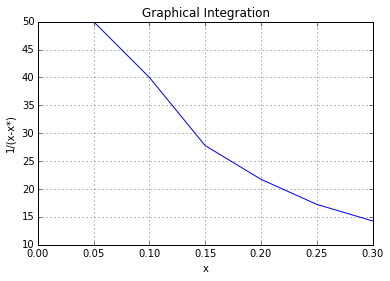Number of theoretical Units:
8.5

Ex10.10:pg-552¶

In :
# Illustration 10.10
# Page: 552

print('Illustration 10.10 - Page: 552\n\n');

# Solution
import math
#****Data****#
B = 1150;# [kg/h]
#*******#

# x and y are taken in weight ratio.
x1_prime = 0.0101;# [Wt. fraction]
xF_prime = 0.0101;# [Wt. fraction]
y2_prime = 0;# [Wt. fraction]
x2_prime = 0.001001;# [Wt. fraction]
y1_prime = 0.0782;# [Wt. fraction]
# From Illustration 10.4:
A = 990.0;# [kg/h]
# At the dilute end:
m1_prime = 0.798;
Value1 = m1_prime*B/A;
# At the concentrated end:
m2_prime = 0.953;
Value2 = m2_prime*B/A;
ValueAv = (Value1*Value2)**0.5;
# From Eqn. 10.116:
# Since y2_prime = 0
Value3 = x2_prime/x1_prime;
NtoR = (math.log((1.0/Value3)*(1-(1/ValueAv))+(1/ValueAv)))/(1-(1/ValueAv));
print"Number of theoretical Unit : ",round(NtoR,1),"\n",
#the answers are slightly different in textbook due to approximation while here answers are precise
Illustration 10.10 - Page: 552

Number of theoretical Unit :  8.6